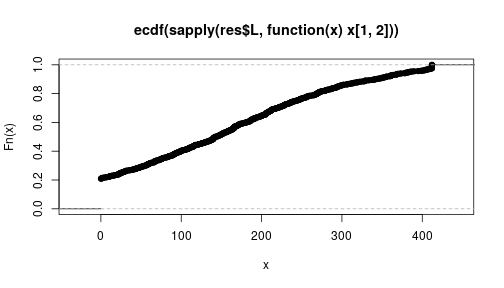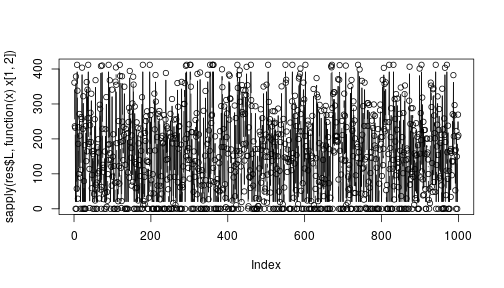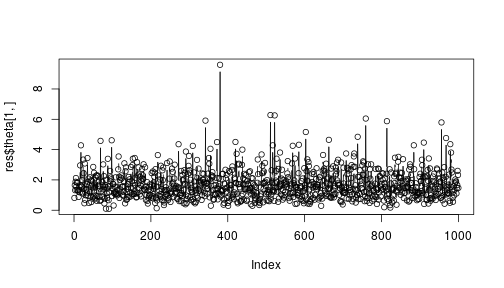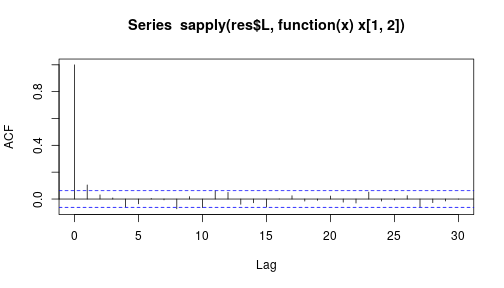An example using the fitness model

This document describe a toy example for the use of the package systemicrisk.

library(systemicrisk)

Suppose we observe the following vector of total liabilities and todal assets.

l <- c(714,745,246, 51,847)
a <- c(872, 412, 65, 46,1208)

The following sets up a model for 5 banks:

lambdaprior=Model.fitness.genlambdaparprior(ratescale=500))

Choosing thinning to ensure sample is equivalent to number of

thin <- choosethin(l=l,a=a,model=mod,silent=TRUE)
## Warning in findFeasibleMatrix_targetmean(l, a, p = u\$p, targetmean =
## mean(genL(model)\$L > : Desired mean degree is less than minimal degree that
## is necessary.

## Warning in findFeasibleMatrix_targetmean(l, a, p = u\$p, targetmean =
## mean(genL(model)\$L > : Desired mean degree is less than minimal degree that
## is necessary.
thin
##  100

Running the sampler to produce 1000 samples.

res <- sample_HierarchicalModel(l=l,a=a,model=mod,nsamples=1e3,thin=thin,silent=TRUE)
## Warning in findFeasibleMatrix_targetmean(l, a, p = u\$p, targetmean =
## mean(genL(model)\$L > : Desired mean degree is less than minimal degree that
## is necessary.

Some examples of the matrics generated are below.

res\$L[]
##           [,1] [,2]     [,3] [,4]     [,5]
## [1,]   0.00000  361 27.49155   46 279.5084
## [2,]  62.50845    0  0.00000    0 682.4916
## [3,]   0.00000    0  0.00000    0 246.0000
## [4,]   0.00000   51  0.00000    0   0.0000
## [5,] 809.49155    0 37.50845    0   0.0000
res\$L[]
##           [,1]     [,2]     [,3]    [,4]     [,5]
## [1,]   0.00000 236.0787 28.91766  0.0000 449.0036
## [2,] 127.77538   0.0000 36.08234  0.0000 581.1423
## [3,]  51.86248   0.0000  0.00000 16.2834 177.8541
## [4,]  51.00000   0.0000  0.00000  0.0000   0.0000
## [5,] 641.36214 175.9213  0.00000 29.7166   0.0000

The sampler produces samples from the conditional distribution of matrix and parameter values given the observed data. To see the posterior distribution of the liabilities of Bank 1 towards Bank 2:

plot(ecdf(sapply(res\$L,function(x)x[1,2])))Diagnostic of the R-output

All the caveats of MCMC algorithms apply. In particular the samples are dependent.

Some automatic diagnostic can be generated via the function diagnose.

diagnose(res)
## Analysis does not consider 5 entries of matrix
## that are deterministic (diagonal elements, row/column sum=0 or forced result).
## All remaining elements of the liabilities matrix have moved during sample run.
## ESS in matrix:
##    Min. 1st Qu.  Median    Mean 3rd Qu.    Max.
##   701.7   891.8  1000.0   940.1  1000.0  1045.5
## ESS in theta:
##    Min. 1st Qu.  Median    Mean 3rd Qu.    Max.
##   607.4   868.1   997.0   986.8  1074.8  1417.5

Trace plots of individual liabilities also shoud show rapid mixing - as seems to be the case for the liabilities of Bank 1 towards Bank 2.

plot(sapply(res\$L,function(x)x[1,2]),type="b")Trace plot of the fitness of bank 1.

plot(res\$theta[1,],type="b")Also, the autocorrelation function should decline quickly. Again, considering the liabilities between bank 1 and bank 2:

acf(sapply(res\$L,function(x)x[1,2]))In this case it decays quickly below the white-noise threshold (the horizontal dashed lines).